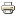# Inheritance of Convexity for Partition Restricted GamesWorking paper
Author/s:
Alexandre Skoda
Issue number:
2016.40
Series:
Documents de travail du Centre d'Economie de la Sorbonne
Publisher:
Centre d'Economie de la Sorbonne
Year:
2016
A correspondence P associates to every subset A ⊆ N a partition P(A) of A and to every game (N,v), the P-restricted game (N,vP) defined by vP(A) = ∑ (F ∈ P(A)) v(F) for all A ⊆ N. We give necessary and sufficient conditions on P to have inheritance of convexity from (N,v) to (N,vP). The main condition is a cyclic intersecting sequence free condition. As a consequence, we only need to verify inheritance of convexity for unanimity games and for the small class of extremal convex games (N,vS) (for any Ø ≠ S ⊆ N) defined for any A ⊆ N by vS(A) = |A ∩ S | − 1 if |A ∩ S | ≥ 1, and vs(A) = 0 otherwise. In particular when (N,v) corresponds to Myerson's network-restricted game inheritance of convexity can be verified by this way. For the Pmin correspondence (Pmin(A) is built by deleting edges of minimum weight in the subgraph GA of a weighted communication graph G, we show that inheritance of convexity for unanimity games already implies inheritance of convexity. Assuming only inheritance of superadditivity, we also compute the Shapley value of the restricted game (N,vP) for an arbitrary correspondence P.
Developed by Paolo Gittoi